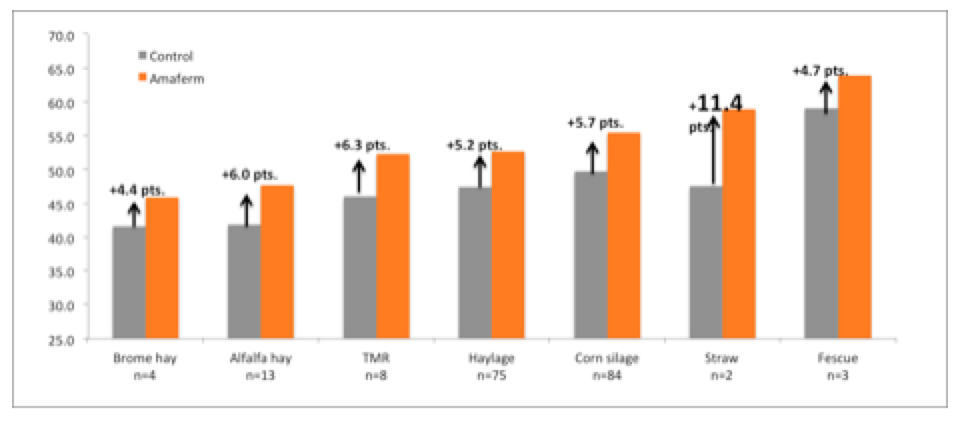# uNDF: New Term, Old Concept, Undigested vs. Indigestible Neutral Detergent Fiber

Fiber digestibility is a critical factor in dairy nutrition. It is involved in many parameters used to balance rations and evaluate forages. It affects DMI and gut fill capacity, as well as total chewing time both eating and ruminating, not to mention milk and milk components production. A new acronym, uNDF is being used in the dairy nutrition lexicon and showing up on some forage analyses relative to NDF digestibility. Here is an explanation of what it is and what it is not.

As part of a small group of researchers working with the CNCPS model determining fiber pools and rates digestion, it became clear that the distinction between undigested fiber and indigestible fiber needed to be clearly defined. For mathematical modeling purposes, iNDF is a required input, however, in the rumen of a dairy cow, iNDF is never approached, but rather uNDF is the more functional predictor of the fiber component. Understanding these distinctions will help clarify differences for both modeling digestion kinetics and evaluating forage quality.

What is uNDF?

uNDF is the undigested NDF residue after fermentation at a given length of time.  It is used to estimate NDF digestibility (NDFD) and is expressed as either a percentage of NDF or percentage of DM. Therefore, uNDF must be accompanied with an indicator of the length of fermentation time; such as 24, 30, 48, 90, 120, 240 h. To be truly correct and a much more accurate predictor, uNDF should also be expressed on organic matter (om) basis to account for residual ash of the forage and any soil contamination of the material. The wet chemistry method of uNDF analysis is a gravimetric procedure and small amounts of mineral/ash can greatly affect the analysis and calculations of digestibility. Therefore, as examples:

A. NDFDom240 equals 100%-uNDFom240

IF “uNDFom240=25% of NDF”.

When expressed on NDF basis, in this case NDFDom240 would be 75%.

B. Grass silage with 52% NDF and a 24h NDFD value of 58% (NDFDom24 =58%)

uNDF24 = 42% on NDF basis (100% – 58% = 42%)

On a DM basis it would be 21.8% of DM (42% NDFDom24 x 52% NDF).

What is iNDF?

uNDF is never really iNDF. Biologically, there is no such thing as iNDF. Indigestible NDF (iNDF) is a theoretical term, unique to each kinetic model for which it is being used to express the totally indigestible portion of forage material. Since digestibility is time dependant, iNDF requires infinite time in order to be determined. Even 240 hours is a long time for the average nutritionist to wait for the results.  As an industry, we have been using the concept of iNDF as an end point of digestion, for the purpose of mathematically calculating the portion of potentially digestible fiber (pdNDF). Thus, rate of digestion of NDF is calculated on only the potentially digestible fraction of NDF.

For modeling purposes we have historically determined lignin as a percent of NDF multiplying by 2.4 to estimate iNDF ( iNDF= (lignin/NDF) x 2.4). We now have a better understanding that this calculation does not accurately estimate a biologically correct iNDF.  Instead, the 240h fermentation residue, uNDFom240, does a better job of predicting the functional undigestible portion. The iNDF fraction as estimated by lignin is highly variable between forage species and is more of a function of the cross linking of phenolic acids than actual lignin.

The nutritional value of uNDF

uNDF is the proportion of NDF that fills the rumen at any given time; a mixture of both the fast and slow pools of pdNDF. Highly digestible fiber will ferment and be physically reduced in particle size to either digest or pass more quickly out of the rumen. The space resulting from digestion and passage of both fast fiber and some portion of the remaining uNDF allows for more DMI. The faster and larger the flux in available rumen space, the greater the intake. The total mass of uNDF residing in the rumen then becomes a baseline of gut fill, limiting this flux. The question becomes, “Is there a maximum and minimum level of uNDF fill in the rumen that limits DMI or, is required to maintain proper rumen health?” We believe that uNDFom240 may be a viable fraction of forage fiber to estimate these limits.

AMAFERM APPLICATION

by Dr. Howard Jensen, BioZyme Inc

The lab value of uNDF should prove useful in more accurately modeling the NDF fraction of the diet.  Knowing the uNDF number will help in better describing the:

• Rates of the fast and slow pools
• Physical fill effect
• Better characterization of different sources of NDF

uNDF is the inverse of the amount of NDF digested at a given time point. AMAFERM Impacts NDFDigestibility on average across all feeds = 17.3% increase.

Incorporating Amaferm in the diet increases the amount of pdNDF that is actually digested at a given end point resulting in more energy available to the animal for increased performance.

IF the lab value for uNDFom24 is 20%, and Amaferm is added to the diet

THEN you could change the uNDFom24 when modeling to reflect the benefit.

Example:

• On AVERAGE, Amaferm research has shown a 17.3% increase in NDF digestion
• 20% x 17.3% = reflects a reduction of 3.46 points
• You could change uNDFom24 value entered from 20% to 16.54% to model the Amaferm value

This helps to formulate a lower cost ration OR explain where the additional milk is coming from.

Abbreviations:

uNDF – the undigested NDF residue after fermentation at a given length of time

NDFD – NDF digestibility

om – Organic matter

DM – Dry matter

iNDF – Indigestible NDF

pdNDF – Potentially digestible NDF